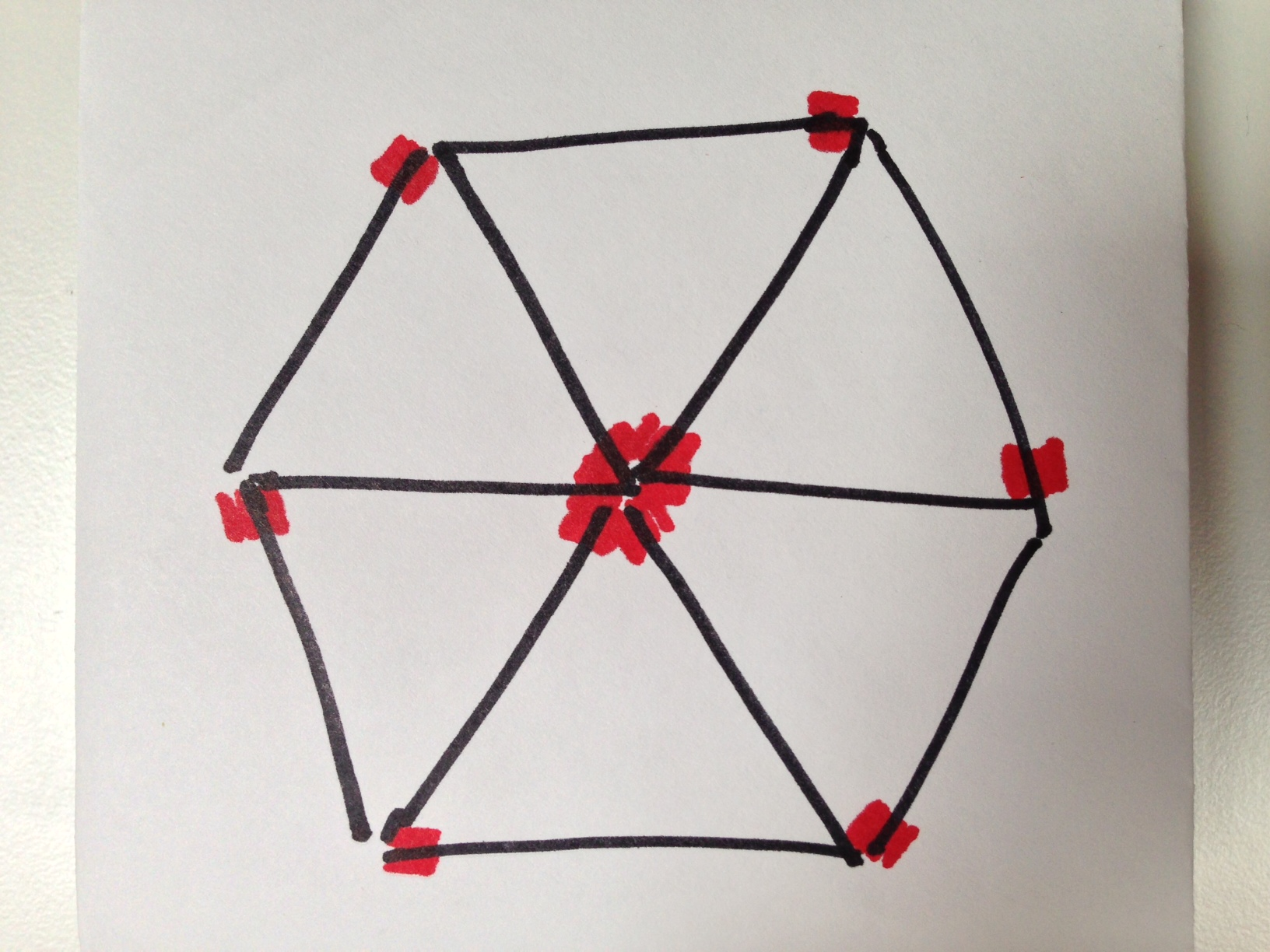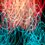# Triangle Matchstick Investigations 2We are investigating the number of matchsticks that we need to form several unit equilateral triangles in 2-D. You should read the first part if you have not already done so.

Let $f(n)$ denote the minimum number of matchsticks that are needed to form $n$ equilateral triangles. In the previous investigation, we tried to determine the value of $f(6)$. From the collective attempts, we arrived at $f(6) = 12$ through the arrangement above. In fact, we have the following table of values:

$\begin{array} { l | l } n & f(n) \\ \hline 1 & 3\\ 2 & 5 \\ 3 & 7 \\ 4 & 9 \\ 5 & 11\\ 6 & 12\\ \end{array}$

Strictly speaking, we don't have any proof that these values hold, other than "We tried our best, but couldn't do better". The accurate statement would be that our attempts show us that $f(6) \leq 12$. There is the slight chance that $f(6) = 11$, because we weren't smart enough to think of something.

Now, let us try and discover more about the function $f(n)$. We have listed out some initial values, but there isn't an immediately observable pattern.

1) What is the value of $f(7), f(8), f(9), f(10)$?

2) Explain why $f(n) \leq f(n+1)$.

3) Is it possible that $f(n) = f(n+1)$? Why, or why not?

4) Show that $f(n+1) \leq f(n) + 2$.
This can be tricky to justify.

5) For what values of $n$ do we have $f(n+1) = f(n) + 1$?

6) Create a table of values of $f(n)$. Can you find any pattern?

As before, we are working in 2-D. If you are brave, go ahead and try the 3-D version. It is quite challenging.Note by Chung Kevin
7 years ago

This discussion board is a place to discuss our Daily Challenges and the math and science related to those challenges. Explanations are more than just a solution — they should explain the steps and thinking strategies that you used to obtain the solution. Comments should further the discussion of math and science.

When posting on Brilliant:

• Use the emojis to react to an explanation, whether you're congratulating a job well done , or just really confused .
• Ask specific questions about the challenge or the steps in somebody's explanation. Well-posed questions can add a lot to the discussion, but posting "I don't understand!" doesn't help anyone.
• Try to contribute something new to the discussion, whether it is an extension, generalization or other idea related to the challenge.

MarkdownAppears as
*italics* or _italics_ italics
**bold** or __bold__ bold
- bulleted- list
• bulleted
• list
1. numbered2. list
1. numbered
2. list
Note: you must add a full line of space before and after lists for them to show up correctly
paragraph 1paragraph 2

paragraph 1

paragraph 2

[example link](https://brilliant.org)example link
> This is a quote
This is a quote
    # I indented these lines
# 4 spaces, and now they show
# up as a code block.

print "hello world"
# I indented these lines
# 4 spaces, and now they show
# up as a code block.

print "hello world"
MathAppears as
Remember to wrap math in $$ ... $$ or $ ... $ to ensure proper formatting.
2 \times 3 $2 \times 3$
2^{34} $2^{34}$
a_{i-1} $a_{i-1}$
\frac{2}{3} $\frac{2}{3}$
\sqrt{2} $\sqrt{2}$
\sum_{i=1}^3 $\sum_{i=1}^3$
\sin \theta $\sin \theta$
\boxed{123} $\boxed{123}$